# Convolutional Neural Networks Training¶

## Introduction¶

Today we will discuss functions we need to override to create a convolutional neural network class based on our NeuralNetworkClassifier class. This will be limited to a single convolutional layer. Let's name the new class NeuralNetworkClassifierCNN. We also assume samples are two-dimensional arrays, like images, with the same number of rows and columns.

We will also introduce how to work with convolutional neural networks in Pytorch, with multiple convolutional layers.

## NeuralNetworkClassifierCNN¶

### __init__¶

The constructor for NeuralNetworkClassifierCNN needs two more arguments, in addition to the usual ones:

• n_inputs: (int) number of components (columns of X) in each sample.
• n_hiddens_per_layer: (list of ints) First int is number of units in the single convolutional layer.
• n_outputs: (int) number of outputs, which equals the number of different classes.
• patch_size: (int) number of rows (equals number of columns) in size of patches. Often called the kernel size.
• stride: (int) number of pixels to shift right and down to produce next patch.
• activation_function='tanh': ('tanh' or 'relu')

To make the right number of weights, we must deal with the convolutional layer first.

# Initialize weights, by first building list of all weight matrix shapes.
n_in = n_inputs
shapes = []
# First build shape of weight matrix for convolutional layer.  Only one allowed.
shapes = [(self.patch_size * self.patch_size + 1, n_hiddens_per_layer)]
input_size = int(np.sqrt(n_inputs))
n_in = ((input_size - self.patch_size) // self.stride + 1) ** 2 * self.n_hiddens_per_layer
for nh in self.n_hiddens_per_layer[1:]:
shapes.append((n_in + 1, nh))
n_in = nh
shapes.append((n_in + 1, self.n_outputs))


### _make_patches¶

We need a new function to convert an input matrix X into patches as discussed previously.

def _make_patches(self, X, patch_size, stride=1):
'''X: n_samples x n_pixels  (flattened square images)'''

X = np.ascontiguousarray(X)  # make sure X values are contiguous in memory

n_samples = X.shape
image_size = int(np.sqrt(X.shape))
n_patches = (image_size - patch_size ) // stride + 1
nb = X.itemsize  # number of bytes each value
new_shape = [n_samples, n_patches, n_patches, patch_size, patch_size]
new_strides = [image_size * image_size * nb,
image_size * stride * nb,
stride * nb,
image_size * nb,
nb]
X = np.lib.stride_tricks.as_strided(X, shape=new_shape, strides=new_strides)
X = X.reshape(n_samples, n_patches * n_patches, patch_size * patch_size)

return X


## use¶

Now that we have _make_patches we know how to modify our use function.

def use(self, X):
'''X assumed to not be standardized. Returns (classes, class_probabilities)'''
# Standardize X
X = (X - self.Xmeans) / self.Xstds
# Convert flattened samples into patches
X_patches = self._make_patches(X, self.patch_size, self.stride)
Ys = self.forward_pass(X_patches)
Y = self.softmax(Ys[-1])
classes = self.classes[np.argmax(Y, axis=1)].reshape(-1, 1)
return classes, Y


### forward_pass¶

As you see, forward_pass has to be modified a bit to handle input as patches. It also must flatten the blurbed image obtained as output of the convolutional layer for use as input to the following fully-connected layer.

def forward_pass(self, X_patches):
'''X assumed already standardized. Output returned as standardized.'''
self.Ys = [X_patches]
for layer_i, W in enumerate(self.Ws[:-1]):
if self.activation_function == 'relu':
self.Ys.append(self.relu(self.Ys[-1] @ W[1:, :] + W[0:1, :]))
else:
self.Ys.append(np.tanh(self.Ys[-1] @ W[1:, :] + W[0:1, :]))
# If convolutional layer, flatten each sample into vector for input to following f
# fully-connected layer.
if layer_i == 0:
self.Ys[-1] = self.Ys[-1].reshape(self.Ys[-1].shape, -1)
last_W = self.Ws[-1]
self.Ys.append(self.Ys[-1] @ last_W[1:, :] + last_W[0:1, :])
return self.Ys


### train¶

The only change needed in train is to create patches from X

X_patches = self._make_patches(X, self.patch_size, self.stride)


and use this form of X as input matrix for optimizer calls, such as

error_trace = optimizer.sgd(self.error_f, self.gradient_f,
fargs=[X_patches, T_indicator_vars], n_epochs=n_epochs,
learning_rate=learning_rate,
verbose=verbose,
error_convert_f=error_convert_f)


### error_f¶

What must we change for error_f?

Nothing! Yay!

### gradient_f¶

However, we do have to do a little work for gradient_f. This is the trickiest part of our convolutional neural network. But, remember, once we move to pytorch, we do not have to write this function!

To the back-propagation loop that steps backwards through the layers we add a special case when we have reached the first layer.

for layeri in range(n_layers - 1, -1, -1):
if layeri == 0:
# Convolutional layer
# delta, backpropagated from a fully-connected layer, has multiple values for each
# convolutional unit, for each application of it to each patch.  We must sum the dE_dWs
# for all of those delta values by multiplying each delta value for each convolutional
# unit by the patch values used to produce the output by the input values for the
# corresponding patch.
# Do this by first reshaping the backed-up delta matrix to the right form.
patch_n_values = X_patches.shape[-1]
n_conv_units = self.n_hiddens_per_layer
delta_reshaped = delta.reshape(-1, n_conv_units)
# And we must reshape the convolutional layer input matrix to a compatible shape.
conv_layer_inputs_reshaped = self.Ys.reshape(-1, patch_n_values)
# Now we can calculate the dE_dWs for the convolutional layer with a simple matrix
# multiplication.
self.dE_dWs[layeri][1:, :] = conv_layer_inputs_reshaped.T @ delta_reshaped
self.dE_dWs[layeri][0:1, :] = np.sum(delta_reshaped, axis=0)
else:
# Fully-connected layers
. . .


## Example use of NeuralNetworkClassifierCNN¶

After you define NeuralNetworkClassifierCNN as a new class that extends NeuralNetworkClassifier, you can use it as in the following example.

Let's make some simple images that are either squares or diamond shapes, like this.

In :
import numpy as np
import matplotlib.pyplot as plt

In :
square = np.array([ * 20,
 * 20,
 * 20,
 * 20,
 * 4 +  * 12 +  * 4,
 * 4 +  +  * 10 +  +  * 4,
 * 4 +  +  * 10 +  +  * 4,
 * 4 +  +  * 10 +  +  * 4,
 * 4 +  +  * 10 +  +  * 4,
 * 4 +  +  * 10 +  +  * 4,
 * 4 +  +  * 10 +  +  * 4,
 * 4 +  +  * 10 +  +  * 4,
 * 4 +  +  * 10 +  +  * 4,
 * 4 +  +  * 10 +  +  * 4,
 * 4 +  +  * 10 +  +  * 4,
 * 4 +  * 12 +  * 4,
 * 20,
 * 20,
 * 20,
 * 20])
square

Out:
array([[0, 0, 0, 0, 0, 0, 0, 0, 0, 0, 0, 0, 0, 0, 0, 0, 0, 0, 0, 0],
[0, 0, 0, 0, 0, 0, 0, 0, 0, 0, 0, 0, 0, 0, 0, 0, 0, 0, 0, 0],
[0, 0, 0, 0, 0, 0, 0, 0, 0, 0, 0, 0, 0, 0, 0, 0, 0, 0, 0, 0],
[0, 0, 0, 0, 0, 0, 0, 0, 0, 0, 0, 0, 0, 0, 0, 0, 0, 0, 0, 0],
[0, 0, 0, 0, 1, 1, 1, 1, 1, 1, 1, 1, 1, 1, 1, 1, 0, 0, 0, 0],
[0, 0, 0, 0, 1, 0, 0, 0, 0, 0, 0, 0, 0, 0, 0, 1, 0, 0, 0, 0],
[0, 0, 0, 0, 1, 0, 0, 0, 0, 0, 0, 0, 0, 0, 0, 1, 0, 0, 0, 0],
[0, 0, 0, 0, 1, 0, 0, 0, 0, 0, 0, 0, 0, 0, 0, 1, 0, 0, 0, 0],
[0, 0, 0, 0, 1, 0, 0, 0, 0, 0, 0, 0, 0, 0, 0, 1, 0, 0, 0, 0],
[0, 0, 0, 0, 1, 0, 0, 0, 0, 0, 0, 0, 0, 0, 0, 1, 0, 0, 0, 0],
[0, 0, 0, 0, 1, 0, 0, 0, 0, 0, 0, 0, 0, 0, 0, 1, 0, 0, 0, 0],
[0, 0, 0, 0, 1, 0, 0, 0, 0, 0, 0, 0, 0, 0, 0, 1, 0, 0, 0, 0],
[0, 0, 0, 0, 1, 0, 0, 0, 0, 0, 0, 0, 0, 0, 0, 1, 0, 0, 0, 0],
[0, 0, 0, 0, 1, 0, 0, 0, 0, 0, 0, 0, 0, 0, 0, 1, 0, 0, 0, 0],
[0, 0, 0, 0, 1, 0, 0, 0, 0, 0, 0, 0, 0, 0, 0, 1, 0, 0, 0, 0],
[0, 0, 0, 0, 1, 1, 1, 1, 1, 1, 1, 1, 1, 1, 1, 1, 0, 0, 0, 0],
[0, 0, 0, 0, 0, 0, 0, 0, 0, 0, 0, 0, 0, 0, 0, 0, 0, 0, 0, 0],
[0, 0, 0, 0, 0, 0, 0, 0, 0, 0, 0, 0, 0, 0, 0, 0, 0, 0, 0, 0],
[0, 0, 0, 0, 0, 0, 0, 0, 0, 0, 0, 0, 0, 0, 0, 0, 0, 0, 0, 0],
[0, 0, 0, 0, 0, 0, 0, 0, 0, 0, 0, 0, 0, 0, 0, 0, 0, 0, 0, 0]])
In :
diamond = np.array([ * 20,
 * 20,
 * 20,
 * 20,
 * 9 +  +  * 10,
 * 8 + [1, 0, 1] +  * 9,
 * 7 + [1, 0, 0, 0, 1] +  * 8,
 * 6 + [1, 0, 0, 0, 0, 0, 1] +  * 7,
 * 5 + [1, 0, 0, 0, 0, 0, 0, 0, 1] +  * 6,
 * 4 + [1, 0, 0, 0, 0, 0, 0, 0, 0, 0, 1] +  * 5,
 * 3 + [1, 0, 0, 0, 0, 0, 0, 0, 0, 0, 0, 0, 1] +  * 4,
 * 4 + [1, 0, 0, 0, 0, 0, 0, 0, 0, 0, 1] +  * 5,
 * 5 + [1, 0, 0, 0, 0, 0, 0, 0, 1] +  * 6,
 * 6 + [1, 0, 0, 0, 0, 0, 1] +  * 7,
 * 7 + [1, 0, 0, 0, 1] +  * 8,
 * 8 + [1, 0, 1] +  * 9,
 * 9 +  +  * 10,
 * 20,
 * 20,
 * 20])
diamond

Out:
array([[0, 0, 0, 0, 0, 0, 0, 0, 0, 0, 0, 0, 0, 0, 0, 0, 0, 0, 0, 0],
[0, 0, 0, 0, 0, 0, 0, 0, 0, 0, 0, 0, 0, 0, 0, 0, 0, 0, 0, 0],
[0, 0, 0, 0, 0, 0, 0, 0, 0, 0, 0, 0, 0, 0, 0, 0, 0, 0, 0, 0],
[0, 0, 0, 0, 0, 0, 0, 0, 0, 0, 0, 0, 0, 0, 0, 0, 0, 0, 0, 0],
[0, 0, 0, 0, 0, 0, 0, 0, 0, 1, 0, 0, 0, 0, 0, 0, 0, 0, 0, 0],
[0, 0, 0, 0, 0, 0, 0, 0, 1, 0, 1, 0, 0, 0, 0, 0, 0, 0, 0, 0],
[0, 0, 0, 0, 0, 0, 0, 1, 0, 0, 0, 1, 0, 0, 0, 0, 0, 0, 0, 0],
[0, 0, 0, 0, 0, 0, 1, 0, 0, 0, 0, 0, 1, 0, 0, 0, 0, 0, 0, 0],
[0, 0, 0, 0, 0, 1, 0, 0, 0, 0, 0, 0, 0, 1, 0, 0, 0, 0, 0, 0],
[0, 0, 0, 0, 1, 0, 0, 0, 0, 0, 0, 0, 0, 0, 1, 0, 0, 0, 0, 0],
[0, 0, 0, 1, 0, 0, 0, 0, 0, 0, 0, 0, 0, 0, 0, 1, 0, 0, 0, 0],
[0, 0, 0, 0, 1, 0, 0, 0, 0, 0, 0, 0, 0, 0, 1, 0, 0, 0, 0, 0],
[0, 0, 0, 0, 0, 1, 0, 0, 0, 0, 0, 0, 0, 1, 0, 0, 0, 0, 0, 0],
[0, 0, 0, 0, 0, 0, 1, 0, 0, 0, 0, 0, 1, 0, 0, 0, 0, 0, 0, 0],
[0, 0, 0, 0, 0, 0, 0, 1, 0, 0, 0, 1, 0, 0, 0, 0, 0, 0, 0, 0],
[0, 0, 0, 0, 0, 0, 0, 0, 1, 0, 1, 0, 0, 0, 0, 0, 0, 0, 0, 0],
[0, 0, 0, 0, 0, 0, 0, 0, 0, 1, 0, 0, 0, 0, 0, 0, 0, 0, 0, 0],
[0, 0, 0, 0, 0, 0, 0, 0, 0, 0, 0, 0, 0, 0, 0, 0, 0, 0, 0, 0],
[0, 0, 0, 0, 0, 0, 0, 0, 0, 0, 0, 0, 0, 0, 0, 0, 0, 0, 0, 0],
[0, 0, 0, 0, 0, 0, 0, 0, 0, 0, 0, 0, 0, 0, 0, 0, 0, 0, 0, 0]])
In :
def draw_neg_image(image, label=''):
plt.imshow(-image, cmap='gray')
plt.xticks([])
plt.yticks([])
plt.title(label)

In :
plt.subplot(1, 2, 1)
draw_neg_image(square, 'square')
plt.subplot(1, 2, 2)
draw_neg_image(diamond, 'diamond');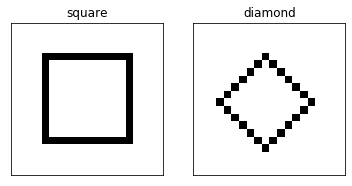Okay, that works. Let's write a function to generate a bunch of images like these, but shifted left to right and up and down to random amounts. This would challenge our fully-connected nets, but maybe not our convolutional net!

In :
def make_images(n_each_class):
'''Make 20x20 black and white images with diamonds or squares for the two classes, as line drawings.'''
images = np.zeros((n_each_class * 2, 20, 20))  # nSamples, rows, columns
radii = 3 + np.random.randint(10 - 5, size=(n_each_class * 2, 1))
centers = np.zeros((n_each_class * 2, 2))
for i in range(n_each_class * 2):
centers[i, :] = r + 1 + np.random.randint(18 - 2 * r, size=(1, 2))
x = int(centers[i, 0])
y = int(centers[i, 1])
if i < n_each_class:
# squares
images[i, x - r:x + r, y + r] = 1.0
images[i, x - r:x + r, y - r] = 1.0
images[i, x - r, y - r:y + r] = 1.0
images[i, x + r, y - r:y + r + 1] = 1.0
else:
# diamonds
images[i, range(x - r, x), range(y, y + r)] = 1.0
images[i, range(x - r, x), range(y, y - r, -1)] = 1.0
images[i, range(x, x + r + 1), range(y + r, y - 1, -1)] = 1.0
images[i, range(x, x + r), range(y - r, y)] = 1.0
T = np.array(['square'] * n_each_class + ['diamond'] * n_each_class).reshape(-1, 1)
return images, T

In :
n_each_class = 100
Xtrain, Ttrain = make_images(n_each_class)
Xtest, Ttest = make_images(n_each_class)
Xtrain.shape, Ttrain.shape, Xtest.shape, Ttest.shape

Out:
((200, 20, 20), (200, 1), (200, 20, 20), (200, 1))
In :
plt.figure(figsize=(10, 3))
for i in range(10):
plt.subplot(2, 10, i + 1)
draw_neg_image(Xtrain[i, :], Ttrain[i, 0])

for i in range(10):
plt.subplot(2, 10, i + 11)
draw_neg_image(Xtrain[i + n_each_class, :], Ttrain[i + n_each_class, 0])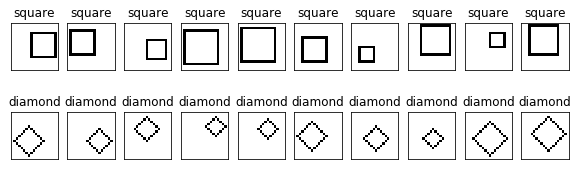In :
import neuralnetworks_A5 as nn


Now we can try to train a CNN!! Our net has been defined to accept two-dimensional input X matrices, so first we must flatten each image.

In :
Ttrain[:10, 0]

Out:
array(['square', 'square', 'square', 'square', 'square', 'square',
'square', 'square', 'square', 'square'], dtype='<U7')
In :
Xtrain = Xtrain.reshape(Xtrain.shape, -1)
Xtest = Xtest.reshape(Xtest.shape, -1)
Xtrain.shape, Xtest.shape

Out:
((200, 400), (200, 400))

Let's try 2 units in the convolutional layer followed by one fully-connected layer of 2 units. And let's use a patch size of 5 and stride of 2.

In :
np.unique(Ttrain)

Out:
array(['diamond', 'square'], dtype='<U7')
In :
nnet = nn. NeuralNetworkClassifierCNN(Xtrain.shape, , len(np.unique(Ttrain)),
patch_size=5, stride=2)
print(nnet)

NeuralNetworkClassifierCNN(400, , 2, 5, 2, 'tanh')

In :
import time

start_time = time.time()
nnet.train(Xtrain, Ttrain, 2000, learning_rate=0.01, verbose=True, method='adam')
classes_train, probs_train = nnet.use(Xtrain)

print(f'took {time.time() - start_time} seconds')
print(f'Train percent correct {100 * np.mean(Ttrain == classes_train)}')

classes_test, probs_test = nnet.use(Xtest)
print(f'Test fraction correct {100 * np.mean(Ttest == classes_test)}')

Adam: Epoch 200 Error=0.99960
took 2.3612639904022217 seconds
Train percent correct 100.0
Test fraction correct 99.5

In :
plt.figure(figsize=(10, 5))
plt.subplot(1, 2, 1)
plt.plot(nnet.error_trace)

Ttest_i = (Ttest == 'diamond').astype(int)

plt.subplot(1, 2, 2)
plt.plot(Ttest_i, 'o-')
plt.plot(probs_test[:, :], '-')
plt.legend(('Ttest', 'Probs Class 1', 'Probs Class 0'))

Out:
<matplotlib.legend.Legend at 0x7f2f95ffea50>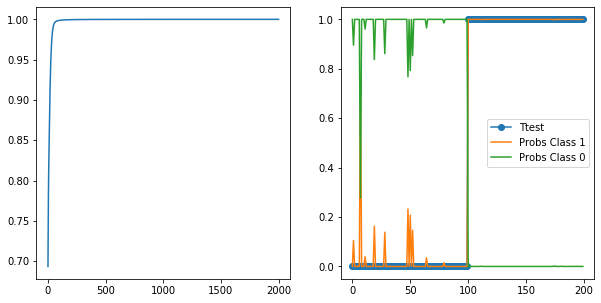Cool. Let's see what our two convolutional units learned. Draw images of their weight matrices.

In :
nnet.Ws.shape

Out:
(26, 2)
In :
conv_W = nnet.Ws[1:, :]  # do not include bias weights

plt.subplot(1, 2, 1)
plt.imshow(conv_W[:, 0].reshape(5, 5))
plt.colorbar()

plt.subplot(1, 2, 2)
plt.imshow(conv_W[:, 1].reshape(5, 5))
plt.colorbar()

Out:
<matplotlib.colorbar.Colorbar at 0x7f2f95fe8290>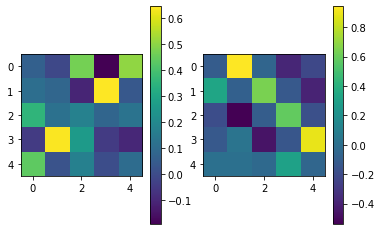Let's repeat, but with two fully-connected layers after the convolutional layer.

In :
nnet = nn. NeuralNetworkClassifierCNN(20 * 20, [4, 10, 10], len(np.unique(Ttrain)),
patch_size=5, stride=2)

start_time = time.time()
nnet.train(Xtrain, Ttrain, 1000, learning_rate=0.01, verbose=True, method='adam')
classes_train, probs_train = nnet.use(Xtrain)

print(f'took {time.time() - start_time} seconds')
print(f'Train percent correct {100 * np.mean(Ttrain == classes_train)}')

classes_test, probs_test = nnet.use(Xtest)
print(f'Test fraction correct {100 * np.mean(Ttest == classes_test)}')

Adam: Epoch 100 Error=0.98569
took 2.34865665435791 seconds
Train percent correct 100.0
Test fraction correct 93.5

In :
plt.figure(figsize=(10, 5))
plt.subplot(1, 2, 1)
plt.plot(nnet.error_trace)

Ttest_i = (Ttest == 'diamond').astype(int)

plt.subplot(1, 2, 2)
plt.plot(Ttest_i, 'o-')
plt.plot(probs_test[:, 0], '-')
plt.legend(('Ttest', 'Probs Class 0', 'Probs Class 1'))

Out:
<matplotlib.legend.Legend at 0x7f2f84b33990>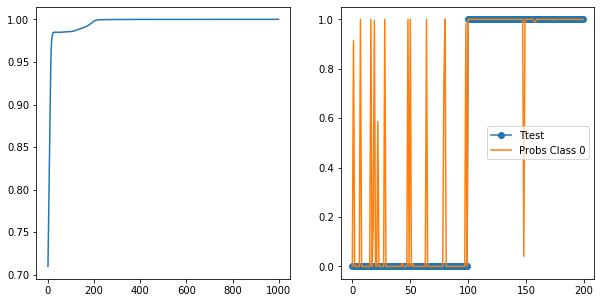Training converges faster, but the generalization to test data not as accurate.

Cool. Let's see what our four convolutional units learned. Draw images of their weight matrices.

In :
nnet.Ws.shape

Out:
(26, 4)
In :
conv_W = nnet.Ws[1:, :]  # do not include bias weights

for uniti in range(4):
plt.subplot(2, 2, uniti + 1)
plt.imshow(conv_W[:, uniti].reshape(5, 5))
plt.colorbar()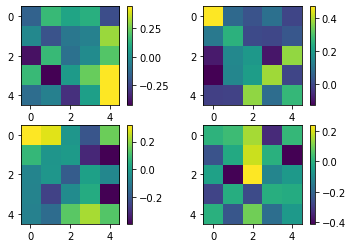How well will our fully-connected net do?

In :
Xtrain.shape, Ttrain.shape

Out:
((200, 400), (200, 1))
In :
# nnet = nn. NeuralNetworkClassifierCNN(20 * 20, [4, 2], len(np.unique(Ttrain)),
#                                        patch_size=5, stride=2)

nnet = nn. NeuralNetworkClassifier(20 * 20, [4, 2], len(np.unique(Ttrain)))

start_time = time.time()
nnet.train(Xtrain, Ttrain, 1000, learning_rate=0.01, verbose=True, method='adam')
classes_train, probs_train = nnet.use(Xtrain)

print(f'took {time.time() - start_time} seconds')
print(f'Train percent correct {100 * np.mean(Ttrain == classes_train)}')

classes_test, probs_test = nnet.use(Xtest)
print(f'Test fraction correct {100 * np.mean(Ttest == classes_test)}')

Adam: Epoch 100 Error=0.97034
took 0.45292043685913086 seconds
Train percent correct 100.0
Test fraction correct 81.0

In :
plt.figure(figsize=(10, 5))
plt.subplot(1, 2, 1)
plt.plot(nnet.error_trace)

Ttest_i = (Ttest == 'diamond').astype(int)

plt.subplot(1, 2, 2)
plt.plot(Ttest_i, 'o-')
plt.plot(probs_test[:, 0], '-')
plt.legend(('Ttest', 'Probs Class 0', 'Probs Class 1'))

Out:
<matplotlib.legend.Legend at 0x7f2f82116550>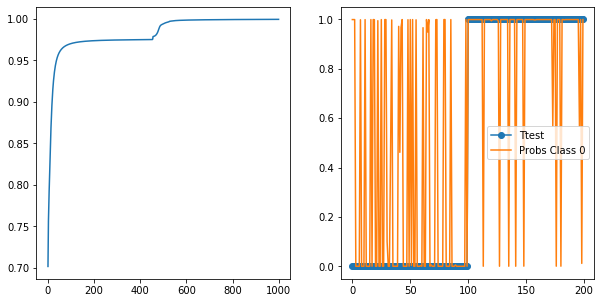In :
first_layer_W = nnet.Ws[1:, :]  # do not include bias weights
print(first_layer_W.shape)

(400, 4)

In :
for uniti in range(4):
plt.subplot(2, 2, uniti + 1)
plt.imshow(first_layer_W[:, uniti].reshape(20, 20))  # notice we have to reshape to the full image size
plt.colorbar()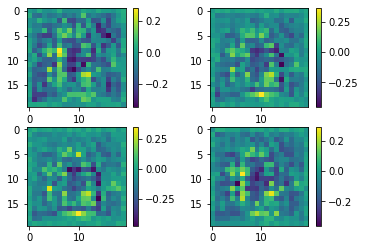In [ ]: